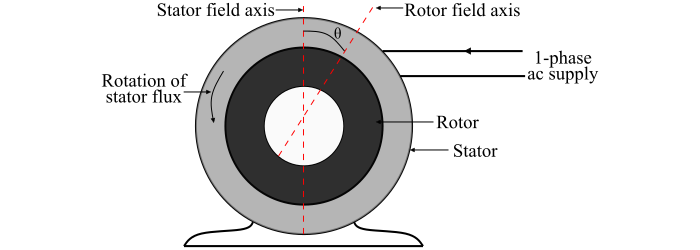# Hysteresis Motor ŌĆō Construction, Operation, Characteristics and Applications

A hysteresis motor is a single-phase synchronous motor whose operating principle is based on the effect of magnetic hysteresis. According magnetic hysteresis, the magnetic flux density in a ferromagnetic material lags behind the magnetising force.

## Construction of Hysteresis Motor

A hysteresis motor consists of a stator and a rotor (see the figure).The stator of the hysteresis motor has a main winding along with an auxiliary or starting winding. When the stator winding is fed from a single-phase supply, it produces a synchronously revolving magnetic field. The revolving magnetic field is accomplished by using a permanent split capacitor type construction of the motor. As a result, both the starting and the main windings of the motor remain connected in the motor circuit during running as well as at starting. The value of the capacitor is so adjusted that it results in a flux revolving at synchronous speed.

The rotor of the hysteresis motor consists of a smooth cylinder of magnetically hard steel, without winding or rotor teeth.

## Operation of Hysteresis Motor

When a single-phase AC supply is connected to the stator winding of the hysteresis motor, a synchronously revolving magnetic field (assumed in counter-clockwise direction) is produced. This revolving magnetic field produced by the stator magnetises the rotor. Due to the effect of magnetic hysteresis, the magnetisation axis of the rotor will lag behind the stator field axis by hysteresis lag angle (θ).

When the rotor is stationary, then the starting torque produced in the motor is given by,

$$\mathrm{τ_{­ØæĀ­ØæĪ} ∝ φ_{­ØæĀ}φ_{­Øæ¤}\:sin ­Ø£ā}$$

Where,

• φ­ØæĀ is the stator flux
• φ­Øæ¤ is the rotor flux.

φ­ØæĀ and φr are constant. When the rotor accelerates, the hysteresis lag angle (θ) remains constant, since the lag angle (θ) depends only upon the hysteresis loop of the rotor and is independent of the rate at which the loop is traversed. Therefore, the motor develops a uniform torque from standstill to the synchronous speed.

Once the synchronism is reached, the motor continues to run at synchronous speed and adjusts its hysteresis lag angle so as to develop the torque required by the load.

## Characteristics of Hysteresis Motor

The characteristics of a hysteresis motor are given as follows −

• Hysteresis motors have uniform torque from standstill to synchronous speed.

• A hysteresis motor can synchronise any load which it can accelerate, no matter how great the inertia of the load.

• A hysteresis motor is inherently quiet and produces smooth rotation of the load.

• In a hysteresis motor, the number of rotor poles are equal to the number of stator poles.

• By changing the number of stator poles through pole-changing connections, a set of synchronous speeds for the motor can be obtained.

## Applications of Hysteresis Motor

The operation of the hysteresis motors is quiet and have the ability to drive the high-inertia loads. Hence, the hysteresis motors are well suited for driving −

• Electric clocks,
• Timing devices,
• Turn-tables, and
• Other precision audio equipment, etc.